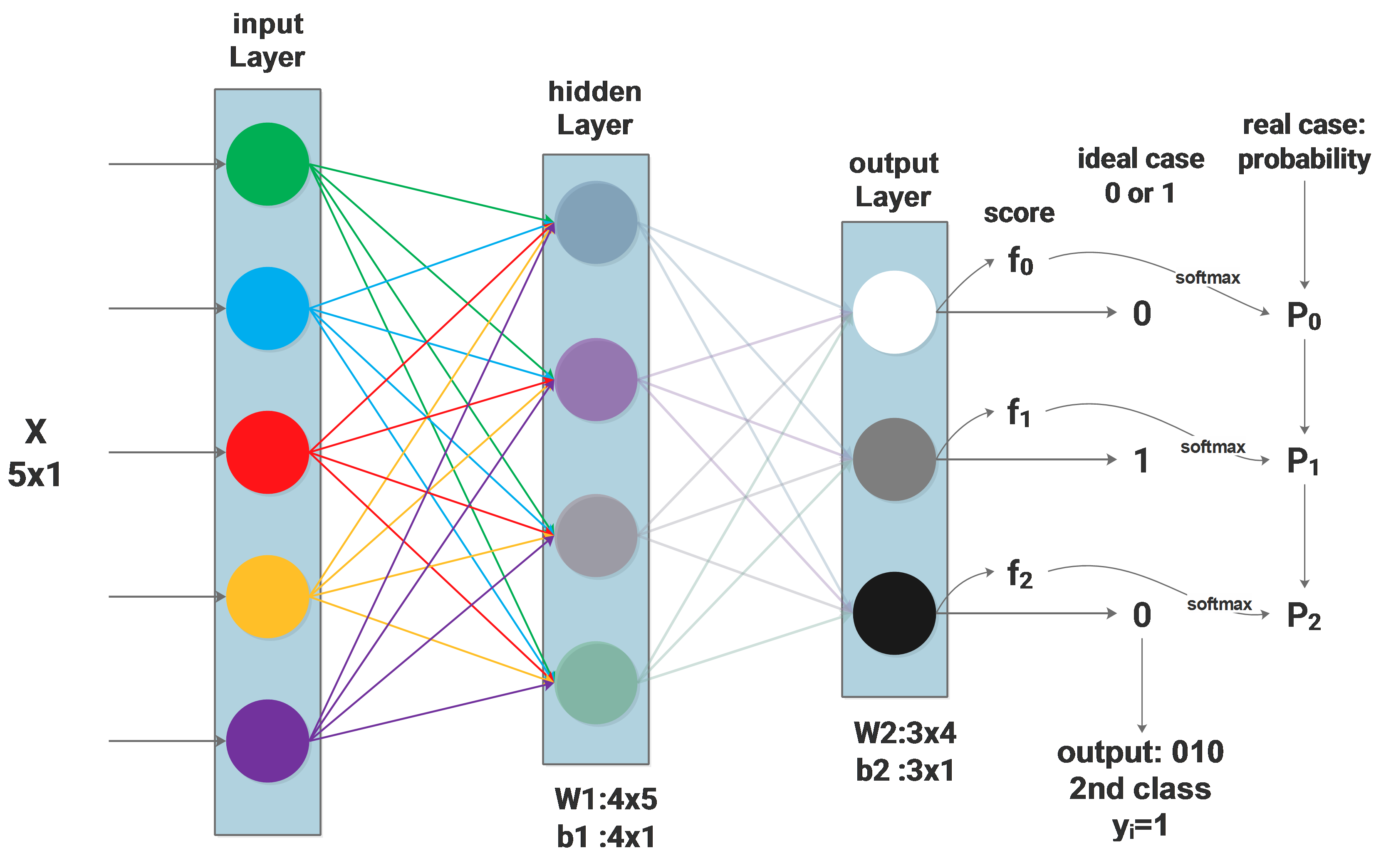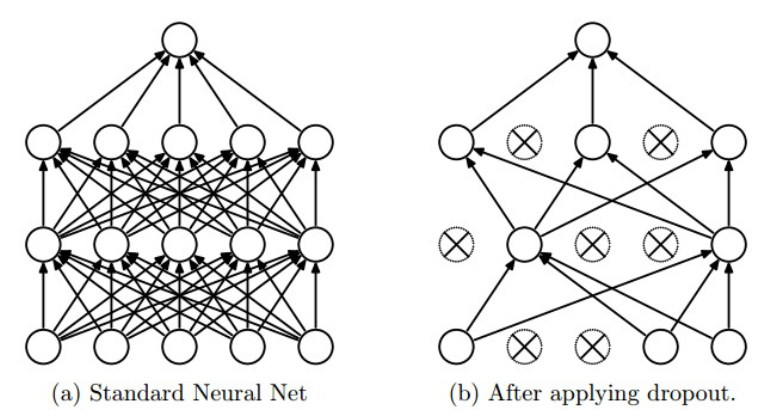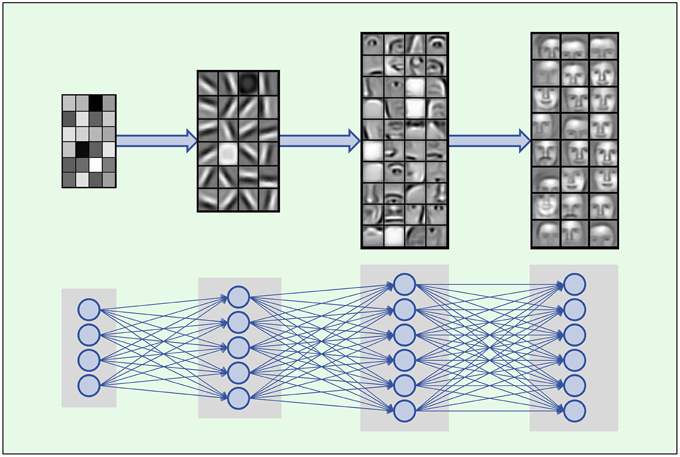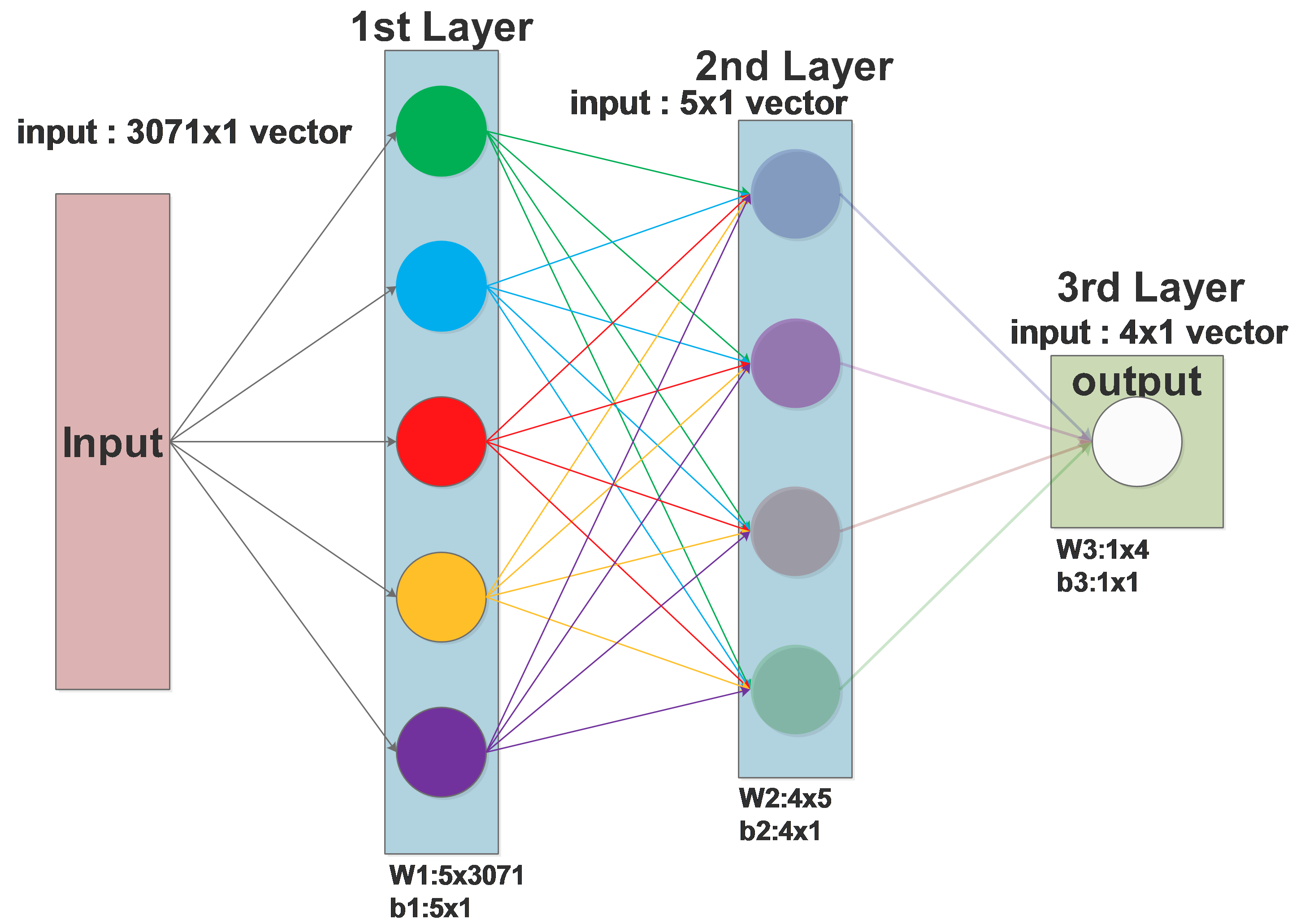# Gaussian Yolo V3 - get 3.5 more mAP and uncertainty with 1% more computation

Uncertainty estimation of the output from a deep neural network has recently become a hot topic, mainly due to Alex Kendall’s PhD thesis Geometry and Uncertainty in Deep Learning for Computer Vision, where he mentioned the uncertainty problems for semantic and geometrical problems in the computer vision field. What is more, it provides us a weighting strategy for the losses of multi-task learning, which is a very practical problem in both the academical and industrial fields. It is highly recommended to take a close look at Alex Kendall’s presentation of his PhD thesis Geometry and Uncertainty in Deep Learning. As a short introduction of Bayesian deep learning, you can also take a look at his blog article Bayesian deep learning - Alex Kendall.

Come to the topic today, the paper published in ICCV 2019 Gaussian YOLOv3: An Accurate and Fast Object Detector Using Localization Uncertainty for Autonomous Driving tries to apply uncertainty estimation for the detection task, and it succeeded. Uncertainty estimation is much more than uncertainty output, it can also be used to construct a loss function and raise the detection accuracy. The paper focused on estimating the uncertainty of the bounding box localization, which means the coordinate of the bounding box center and its width and height. By using the uncertainty estimation, the paper managed to achieve 3.5 more mAP than the original YoloV3 with 1% more computational cost, what is more, the implementation is also quite straight forward. This blog will provide a in-depth explaination of this paper.

# Graph Optimization 4 - g2o introduction - GPS odometry

In graph optimization 1 to 3, the math is introduced. Now we can make some hands on practice on programming. The most famous used graph optimization library is g2o due to its good performance in ORB-SLAM. g2o also has its well known drawback - not well commented, not easy to understand. What’s more, most of the tutorials are based on the original sample examples, when you want to make your own vertex or edge, you will again be lost.

This introduction will be based on an easy to understand graph optimization problem with a customized edge implementation.

# Modelling the GPS based odometry

## Model as graph

The problem is quite easy: we have a vehicle moving around, we use a GPS to measure its 3D absolute positions, by making some rough guesses as initialization, we want to estimate the vehicle’s position based on the GPS’ measurements.

In a SLAM system, we usually want to fuse different sensors, what’s discussed most are fusing camera and IMU, which is also the problem setup for g2o examples. Fusing GPS information is rarely touched, actually GPS sensor fusion is easier to understand. It can be modeled as the following diagram:# Graph Optimization 3 - Optimization Update

From the last article, we get the following negative log likelihood function as our optimization target:

$F(x)=\sum_{ij}{e_{ij}(x)^T\Omega_{ij}e_{ij}(x)}$

The optimization problem turns to be:

$x^*=\arg\min_xF(x)$

This article will explain how can this optimization problem be solved using Gauss-Newton method.

# Graph Optimization 2 - Modelling Optimization Target

Usually, all graph optimization papers or tutorials start from raising the optimization problem: minimizing the following function:

$F(x)=\sum_{ij}{e_{ij}(x)^T\Omega_{ij}e_{ij}(x)}$

This article will explain where this optimization comes from and why it is related to gaussian noise.

## Maximum Likelihood Estimation with Gaussian noise

The probabilistic modeling of graph optimization is based on the Maximum Likelihood Estimation(MLE) algorithm.

The case we use for this article will also be the one we used in the last article:# SVD, PCA and Least Square Problem

### The idea behind PCA

In the field of machine learning, PCA is used to reduce the dimension of features. Usually we collect a lot of feature to feed the machine learning model, we believe that more features provides more information and will lead to better result.

But some of the features doesn’t really bring new information and they are correlated to some other features. PCA is then introduced to remove this correlation by approximating the original data in its subspace, some of the features of the original data may be correlated to each other in its original space, but this correlation doesn’t exit in its subspace approximation.

Visually, let’s assume that our original data points $$x_1...x_m$$ have 2 features and they can be visualized in a 2D space:

# NN Backpropagation

### The case we are handling: a 2 layers network# NN Softmax loss function

### Background:the network and symbols

Firstly the network architecture will be described as:# NN Dropout

### Dropout regularization

Dropout is a commonly used regularization method, it can be described by the diagram below: only part of the neurons in the whole network are updated. Mathematically, we apply some possibility $$p$$(we use 0.5) to a neuron to keep it active or keep it asleep:# NN Initialization

### Weight Initialization

All-Zero Initialization

It is easy to think that we set all the weights to be zero, but it’s terribly wrong, cause using all zero initialization will make the neurons all the same during the backpropagation update. We don’t need so many identical neurons. Actually, this problem always exists if the weights are initialized to be the same.

Small random values

One guess to solve the problem of all-zero initialization is setting the weights to be small random values, such as $$W=0.01*np.random.randn(D,H) ​$$ . It is also problematic because very small weights cause very small updates and the update values become smaller and smaller during the backpropagation. In the deep network, this problem is very serious as you may find that the upper layers never update.

# Discriminatively Trained Part Based Models notes

The LSVM (SVM with latent variable) is mostly used for human figure detection, it is very efficiency because it puts the human figure’s structure into consideration: a human figure has hands, head and legs. The LSVM models the human figure structure with 6 parts, and the position of these 6 parts are latent value.

The basic logic is sliding a window on the image, for every position we get a small image patch, by scoring this image patch we can predict whether this image patch contains a human figure or not.

### Defining the score function

Anyway, the first thing to do: defining a score function:

# Structural SVM with Latent Variables Notes

Structural SVM is a variation of SVM, hereafter to be refered as SSVM

### Special prediction function of SSVM

Firstly let’s recall the normal SVM’s prediction function:

$f(x)=sgn((ω\cdot x)+b)$

ω is the weight vector，x is the input，b is the bias，$$sgn$$ is sign function，$$f(x)$$ is the prediction result.

On of SSVM’s specialties is its prediction function：

$f_ω (x)=argmax_{y∈Υ} [ω\cdot Φ(x,y)]$

y is the possible prediction result，Υ is y’s searching space，and Φ is some function of x and y.Φ will be a joint feature vector describes the relationship between x and y

Then for some given $$\omega$$, different prediction will be made according to different x.

# Lecture notes of LSTM's Inventor Sepp Hochreiter

Sepp Hochreiter was graduated from Technische Universität München, LSTM was invented when he was in TU and now he is the head of Institute of Bioinformatics, Johannes Kepler University Linz.

Today he comes by Munich and gives a lecture in Fakultät Informatik.

At first, Hochreiter praised how hot is Deep Learning (to be referred as DL) around the world these days, especially LSTM, which is now used in the new version of google translate published a few days ago. The improvements DL made in the fields of vision and NLP are very impressive.

Then he starts to tell the magic of DL, taking face recognition as an example, the so-called CNN (Convolution Neuro Networks):# NN Preprocessing

### Centering and Scaling# NN Architecture# NN:one neuron

### Simple NeuronThe above diagram shows a neuron in NN, it simulates a real neuron:

it has inputs: $$x_{0},x_{1}\dots x_{i}$$

it has weights for each inputs: $$\omega_{0},\omega_{1}\dots \omega_{i}$$: weight vector

it has bias $$b$$

it has a threshold for the “activation function”

# SVM:multi-class SVM regularization

### Review

For the ith sample $$(x_i,y_i)$$ in the training set, we have the following loss function:

$L_i= \sum_{j≠y_i}max(0,w_j^T\cdot x_i−w_{y_i}^T\cdot x_i+Δ)$

$$w_j^T\cdot x_i$$ is the score classifying $$x_i$$ to class j，and $$w_{y_i}^T\cdot x_i$$ is the score classifying correctly(classify to class $$y_i$$)，$$\omega_i$$ is the $$j$$th row of $$W$$.

### Problem

Problem 1:

Considering the geometrical meaning of the weight vector $$\omega$$, it is easy to find out that $$\omega$$ is not unique, $$\omega$$ can change in a small area and result in the same $$L_i$$.

Problem 2:

It the values in $$\omega$$ is scaled, the loss computed will also be scaled by the same ratio. Considering a loss of 15, if we scale all the weights in $$\omega$$ by 2, the loss will be scaled to 30. But this kind of scaling is meaningless, it doesn’t really represent the loss.

# PCA and Face Recognition - Eigen Face

PCA (Principal component analysis), just as its name shows, it computes the data set’s internal structure, its “principal components”.

Considering a set of 2 dimensional data, for one data point, it has 2 dimensions $$x_1$$ and $$x_2$$ . Now we get n such data points . What is the relationship between the first dimension $$x_1$$ and the second dimension $$x_2$$ ? We compute the so called covariance:

$cov(x_1,x_2)=\frac\sum_{i=1}^n{(x_1^i-\overline{x_1})(x_2^i-\overline{x_2})} }{n-1$

the covariance shows how strong is the relationship between $$x_1$$ and $$x_2$$. Its logic is the same as Chebyshev’s sum inequality:

# HoG Feature

HoG (Histograms of Oriented Gradients) feature is a kind of feature used for human figure detection. At an age without deep learning, it is the best feature to do this work.

Just as its name described, HoG feature compute the gradients of all pixels of an image patch/block. It computes both the gradient’s magnitude and orientation, that’s why it’s called “oriented”, then it computes the histogram of the oriented gradients by separating them to 9 ranges.

One image block (upper left corner of the image) is combined of 4 cells, one cell owns a 9 bins histogram, so for one image block we get 4 histograms, and all these 4 histograms will be flattened to one feature vector with a length of 4x9. Compute the feature vectors for all blocks in the image, we get a feature vector map.

Taking one pixel (marked red) from the yellow cell as an example: compute the $$\bigtriangledown_x$$ and $$\bigtriangledown_y$$ of this pixel, then we get its magnitude and orientation(represented by angle). When calculating the histogram, we vote its magnitude to its neighboring 2 bins using bilinear interpolation of angles.

Finally, when we get the 4 histograms of the 4 cells, we normalize them according to the summation of all the 4x9 values.

The details are described in the following chart:

# Linear Classification

### Loss Function

Now we want to solve a image classification problem, for example classifying an image to be cow or cat. The machine learning algorithm will score a unclassified image according to different classes, and decide which class does this image belong to based on the score. One of the keys of the classification algorithm is designing this loss function.

Map/compute image pixels to the confidence score of each class

Assume a training set:

$(x_i,y_i)$

$$x_i$$ is the image and $$y_i$$ is the corresponding class

i∈1…N means the traning set constains N images

$$y_i$$∈1…K means there are K image categories

So a score function maps x to y:

$f(x_i,W,b)=W\cdot x_i+b$

In the above function, each image $$x_i$$ is flattend to a 1 dimention vector

If one image’s size is 32x32 pixels with 3 channels

$$x_i$$ will be a 1 dimention vector with the length of D=32x32x3=3072 Parameter matrix W has the size of [KxD], it is often called weights b of size [Kx1] is often called bias vector In this way, W is evaluating $$x_i$$’s confidence score for K categories at the same time

# Goodbye Mobis

After two years of happy and constructive time in camera team, I got some homesick, I decided to go back to my 2nd hometown, Munich.

The days in Mobis are amazing. I learned so many new things and read so many papers that I can not even believe it, especially when I am cleaning them for the moving, Luka’s enthusiasm of new technologies motivated me to track the trend, which at end, benefits the design of our technical roadmap. The lessons I learned from Luka worth dual, maybe triple master degrees, one for geometry, one for deep learning and one for management, together with the lessons learned from everyone of camera team, I will definitely give myself an honored PhD title, awarded by camera team.

Before I joined camera team, I only have friends from few countries, now I have worked with the talents from so many countries and cultural backgrounds, the experience is unbelievably great. I got to understand so many cultures and religions, vividly, in person. My view of the world expanded so much here that I understand the world much more than before. The different cultures didn’t shock we, the diversity and the harmony of our team do amazed me. I would give special thanks to Thusita for constructing such a great team.

Conflicts and different technical views do exist, which is always helpful for us to regularize each other, I would consider these more as a kind of unsupervised learning. Thank you everyone for your tolerance on me, there are so many s**ty words came from my mouth and I am quite sure some of you guys definitely got ear pollution or even ear cancel.

I feel sad that I am leaving the camera team at this specific time, by doing good work, we win the trust from MTCK, we are working on more and more projects, we are moving to a bigger place and expanding further, last but not least, we just got a new lunch supplier!

It has been a great pleasure working with you all and I believe that we will have successful collaboration in the future!